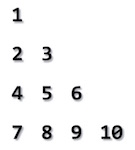# Floyd's Triangle Program In C

Floyd's triangle, named after Rober Floyd, is a right angled triangle, which is made using natural numbers. It starts from 1 and consecutively selects the next greater number in sequence.We shall here learn how to print floyd's triangle using C programming language.

## Algorithm

Algorithm should look like this −

```Step 1 - Take number of rows to be printed, n.
Step 2 - Make outer iteration I for n times to print rows
Step 3 - Make inner iteration for J to I
Step 3 - Print K
Step 4 - Increment K
Step 5 - Print NEWLINE character after each inner iteration
Step 6 - Return
```

## Pseudocode

We can derive a pseudocode for the above mentioned algorithm, as follows −

```procedure floyds_triangle

FOR I = 1 to N DO
FOR J = 1 to I DO
PRINT K
INCREMENT K
END FOR
PRINT NEWLINE
END FOR

end procedure
```

## Implementation

Implementation of right triangle in C is as follows −

```#include <stdio.h>

int main() {
int n,i,j,k = 1;

n = 5;

for(i = 1; i <= n; i++) {
for(j = 1;j <= i; j++)
printf("%3d", k++);

printf("\n");
}

return 0;
}
```

The output should look like this −

```  1
2  3
4  5  6
7  8  9 10
11 12 13 14 15
```
patterns_examples_in_c.htm